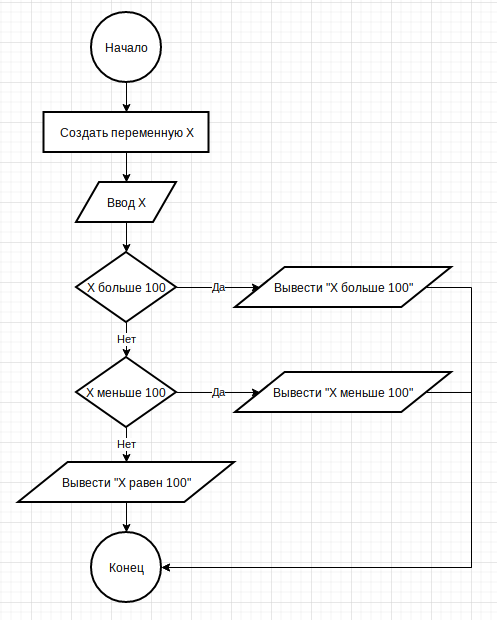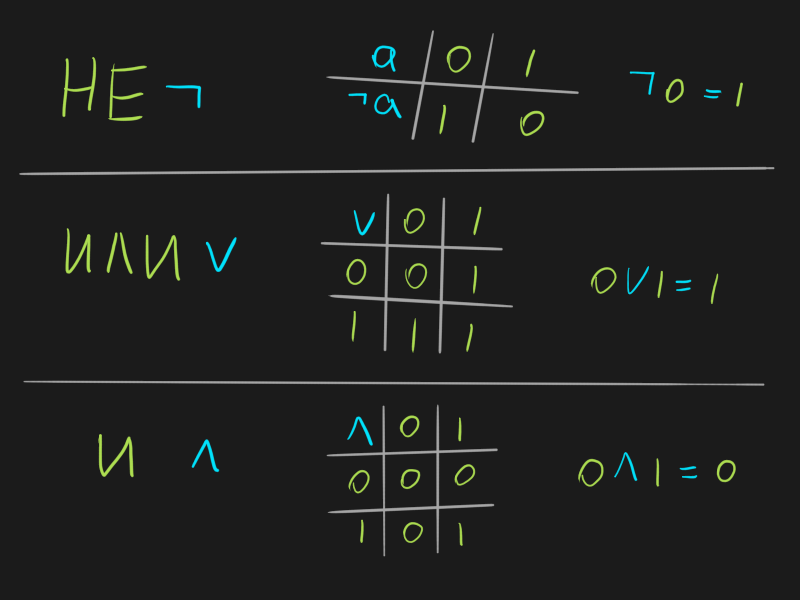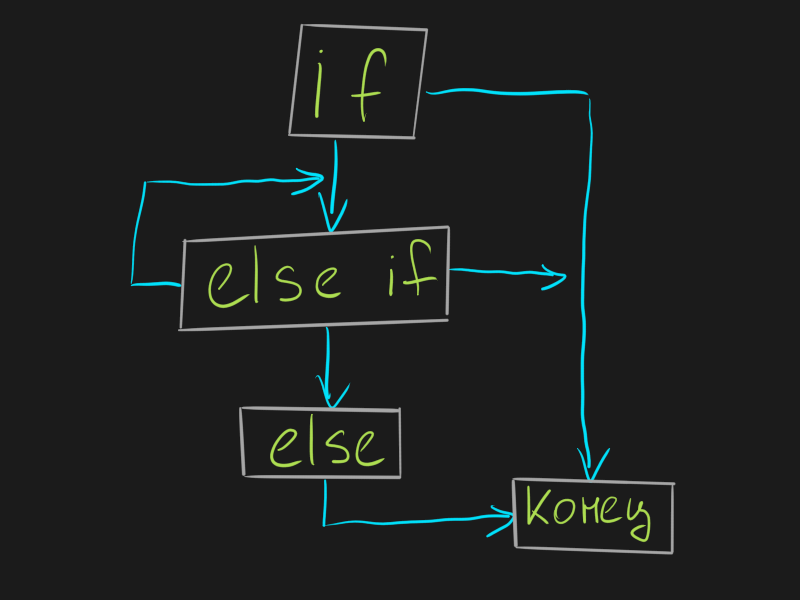+---------------------------+
|.-------------------------.|
|| kee_reel@blog:~/en \$ cd ||
|| linux c                 ||
||                         ||
||                         ||
|.-------------------------.|
+-::---------------------::-+
.---------------------------.
// /oooooooooooooooooooooo\\ \\
// /oooooooooooooooooooooooo\\ \\
//-------------------------------\\
\\-------------------------------//

# C. Conditions

A condition is a mechanism that allows an action to be performed only if a certain condition is met.

Here is an example of a condition definition:

``````int x;
scanf("%d", &x);
if(x > 100)
{
printf("The number %d is greater than 100\n", x);
}
else if(x == 100)
{
printf("The number %d is 100\n", x);
}
else
{
printf("The number %d is less than 100\n", x);
}
``````

To better understand what’s going on, I’ll draw a flowchart.

A flowchart is just a graphic diagram that describes some kind of algorithm. With its help, you can describe the algorithm itself without bothering with writing a program in any programming language.

Algorithm is a sequence of actions.

Here is the block diagram for the code above:Made with draw.io online flowchart editor

In flowcharts, everything is very simple - you just need to follow the arrows from “beginning” to “end” and answer questions if they are asked. The questions in these diagrams are the conditions I’m talking about.

I hope this explained the meaning of the conditions.

Now I will explain why questions/conditions can only be answered with “yes” or “no”…

# Boolean and comparison operations

There is a branch of mathematics called Boolean Algebra. From it, the following concepts came to programming:

• The true value, which is denoted by the number 1
• The value is false, which is denoted by the number 0
• NOT operator (¬) – this unary operator inverts a value
• OR operator (∨) – this binary operator returns true if at least one of its operands is true
• AND operator (∧) – this binary operator returns true if all operands are true

Truth tables are used to describe the various results of Boolean operations:### Boolean operations

In the C language, these concepts are denoted as follows:

• true: any integer or real value not equal to 0
• false: integer or real value equal to 0
• NOT: ! (exclamation point symbol)
• OR: || (two vertical slashes)
• AND: && (two ampersand characters)

Although the value true in C can be expressed as any number other than 0, programmers usually use the value 1.

The operations NOT, OR, AND always return either 0 (false) or 1 (true).

Here is an example of using these operations:

``````// I use the char type so as not to take up extra memory - after all, only the values \u200b\u200bof 0 and 1 are needed
char thisIsTrue = 1;
char thisIsFalse = 0;

// Negate true
char result = !thisIsTrue;
printf("!1 = %d\n", result); // !1 = 0

// Negate with value false
result = !thisIsFalse;
printf("!0 = %d\n", result); // !0 = 1

// OR operation
result = thisIsFalse || thisIsTrue;
printf("0 || 1 = %d\n", result); // 0 || 1 = 1
result = thisIsFalse || 0;
printf("0 || 0 = %d\n", result); // 0 || 0 = 0

// Operation I
result = thisIsFalse && thisIsTrue;
printf("0 && 1 = %d\n", result); // 0 && 1 = 0
result = 1 && thisIsTrue;
printf("1 && 1 = %d\n", result); // 1 && 1 = 1

// Don't forget that everything except 1 is also considered true!
result = !51;
printf("!51 = %d\n", result); // !51 = 0
result = 0 || 522;
printf("0 || 522 = %d\n", result); // 0 || 552 = 1
result = 62.2 && 23;
printf("62.2 && 23 = %d\n", result); // 62.2 && 23 = 1
``````

#### Tricky job interview

Due to the fact that Boolean operations always return either 1 or 0, programmers have come up with a tricky task that they like to ask at job interviews.

Now I will show it, explain it, and you will never fail on it :)

At the interview, they give you the following code:

``````int a = 42;
a = !!a;
printf("%d", a);
``````

And they ask - “What will the program output?”.

Let’s break down the “!!a” operation into separate execution steps:

• Do the rightmost “!” first: !a -> !42 -> 0
• Then execute the remaining “!” to what happened after the previous stage: !0 -> 1

Answer at the interview: 1 will be displayed.

### Comparison operations

Even in the C language there are comparison operations that are no different from those in mathematics:

• Equals: ==
• Not equal to: !=
• More: >
• Greater than or equal: >=
• Less than: <
• Less than or equal to: <=

These operations, like boolean operations, return a boolean value of 0 (false) or 1 (true).

Here is an example of using these operations:

``````char ten = 10;
char hundred = 100;
// Equals
char result = ten == hundred;
printf("10 == 100 = %d\n", result); // 10 == 100 = 0
// Not equal
result = ten != hundred;
printf("10 != 100 = %d\n", result); // 10 != 100 = 1
// More
result = ten > hundred;
printf("10 > 100 = %d\n", result); // 10 > 100 = 0
// Less or equal
result = ten <= hundred;
printf("10 <= 100 = %d\n", result); // 10 <= 100 = 1
``````

### Combine boolean, comparison and arithmetic operations

Often you will need to combine several operators with each other to describe some kind of logic.

Here is an example of a task: “Check that the number is even and less than 100”. Here’s how I would write:

``````int x;
scanf("%d", &x);
char isOk = !(x % 2) && x < 100;
printf("Number fits (1 - yes, 0 - no): %d\n", isOk);
``````

What is “!(x % 2) && x < 100”? it

• x % 2 – the remainder of dividing a number by 2: if the number is even, the remainder will be 0, and if it is odd, it will be 1
• !(x % 2) – boolean operation NOT on the result of the expression (x % 2): if the result is 0 (even), then the result is 1
• x < 100 – comparing a number with 100: if the number is less, then the result will be 1, and if greater than or equal, then 0
• !(x % 2) && x < 100 – boolean AND operation on the results of the left and right sides: if the number is even AND less than 100, then 1 will be returned

For clarity, I will substitute the specific number 42, and simplify it down to the result:

``````!(42 % 2) && 42 < 100
!(0) && 1
1 && 1
``````

You can also substitute specific numbers to make it easier to understand the logic inside complex operations.

# Back to where we started

…that’s why, the answer to the condition can only be the answer “yes” or “no” :)

Once again I will write the code with the condition from the beginning of the article:

``````int x;
scanf("%d", &x);
if(x > 100)
{
printf("The number %d is greater than 100\n", x);
}
else if(x == 100)
{
printf("The number %d is 100\n", x);
}
else
{
printf("The number %d is less than 100\n", x);
}
``````

An expression is written in the condition that returns the value 0 (false) or 1 (true). The following curly braces contain a block of code that will be executed if the expression evaluates to true:

``````// if(expression)
if(x > 100)
// Start of the block
{
printf("The number %d is greater than 100\n", x);
}
// End of block
``````

If the expression inside is equal to true (not equal to 0), then we execute the block of code inside this condition, and finish.

If the expression inside is false, then you need to check if there is an else construct after this if:

• If there is no else, we are done
• If there is else – go to the next condition

Let’s assume that the first condition was not fulfilled for us - now we have moved on to the next one. Here is the same if, but with a different condition:

``````else if(x == 100)
{
printf("The number %d is 100\n", x);
}
``````

We check if there is an else after it - yeah, there is.

There is no longer a condition, so we just execute the block of code inside:

``````else
{
printf("The number %d is less than 100\n", x);
}
``````

An else construct without conditions:

• Optional (you can leave only if)
• Must be placed after the if construct
• After it, you can not use other if or else constructs

It turns out such a cycle if in nature:# Nested conditions

Short but important – inside any condition there can be other conditions.

Look:

``````int x;
scanf("%d", &x);
if(x != 100)
{
if(x > 100)
{
printf("The number %d is greater than 100\n", x);
}
else
{
printf("The number %d is less than 100\n", x);
}
}
else
{
printf("The number %d is 100\n", x);
}
``````

The result of executing this code is identical to the result of executing the code from the previous section - it’s just that I moved two conditions under the first condition, which changed the internal logic of the program.

In your practice, situations will periodically arise when it will be difficult for you to correctly compose a construction from conditions - in this case, draw on paper a flowchart of the algorithm that you want to write.

# Ternary operator

Sometimes, if the condition is small, it can be written using the ternary operator “?:”.

Ternary because it has 3 operands: A ? B : C Addition, for example, is a binary operator, there are 2 operands: A + B.

Let’s look at an example of such a task:

• Two integer variables X and Y are created
• The value of the variable X is entered from the keyboard
• If X is greater than 100, then Y = X * X
• If X is 100 then Y = X
• In other cases (X is less than 100), then Y = X * 2

Here’s how I would write it with an if:

``````int X, Y;
scanf("%d", &X);
if(x > 100)
{
Y = X * X;
}
else if(x == 100)
{
Y = X;
}
else
{
Y = X * 2;
}
printf("Y = %d\n", Y);
``````

How about using the ternary operator “?:”

``````int X, Y;
scanf("%d", &X);
// If x > 100, then X * X will be returned in Y
// Else we'll go into this expression ( x == 100 ? X : (X * 2) )
// It has the same meaning - if x == 100, then X will be returned
// Else return X * 2
Y = x > 100 ? (X * X) : ( x == 100 ? X : (X * 2) );

printf("Y = %d\n", Y);
``````

With this operator, it becomes more difficult to read, but the record is shorter. Use it as needed.

# Conditional statement switch case

In cases where you have a variable that:

• Can take a limited set of values
• You need to do different actions for different values

Use switch case:

``````int day_of_week;
printf("Enter the number of the day of the week: ");
scanf("%d", &day_of_week);
switch(day_of_week) {
case 1:
printf("Monday\n");
break;
case 2:
printf("Tuesday\n");
break;
case 3:
printf("Wednesday\n");
break;
case 4:
printf("Thursday\n");
break;
case 5:
printf("Friday\n");
break;
case 6:
printf("Saturday\n");
break;
case 7:
printf("Sunday\n");
break;
default:
printf("There is no such day\n");
}
``````

Here, in the switch, an expression is written that will be compared with the values in the described cases.

If the specified expression does not fit any case, then we will fall into default. default is optional and can be omitted.

The code for each case must be written between “case X:” and “break;”.

#### A simple task on conditions and expressions inside them

An integer is entered from the keyboard. In response, the program displays one of the answers:

• “Even” – if the number is even
• “Odd” – if the number is odd
• “The answer to the ultimate question of life, the universe and all that” – if this number is 42

#### Block diagram

Draw on paper or in an online editor a flowchart for the task you just did (you can rely on the flowchart at the beginning of the article).

# Conclusion

In total, you have learned:

• Flowcharts
• Boolean values and operators
• Truth tables
• Answering a tricky job interview
• Comparison operators
• Conditions
• Nested conditions

This is a very important stage in your development as a programmer. Conditions are used everywhere, and if you understand them now, then you can easily transfer this knowledge to other programming languages in the future.

If anything - write, I will help and try to explain better.

Next, we’ll look at the main workforce of your programs – loops.